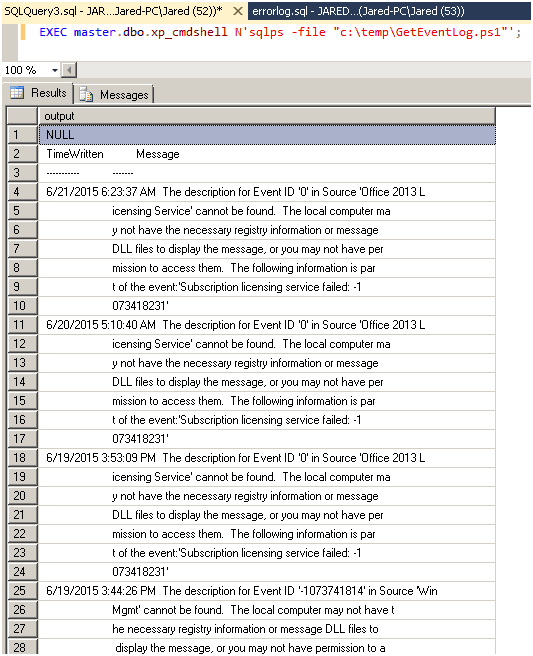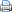In Part I of Check for Errors First, we discovered how to query the error logs, and filter out the noise to find meaningful errors that could contribute to performance and stability issues. In Part II of Check for Errors First, we explored how to look for errors in the system trace which runs continually whenever SQL Server starts. Now we are going to pull the Windows Event logs so that we will have a complete understanding of the system logging that could help define any issues on the current system.

There are a few moving parts to this solution, but I will break them up and explain each item as we go through the solution. In this solution we will be leveraging powershell, so lets start with the powershell code and then we can move on to the T-SQL process that will call our sqlps script.

We will save the above code as: “C:\Temp\GetEventLog.ps1”.  The first part of the code “Get-EventLog -Logname Application -EntryType Error” – states that it is calling the Application Log in the Event viewer where there is an entry type of “Error” (There are several informational records recorded that is noise, we want just the errors so we are removing the noise). The next part, “-After (Get-Date).adddays(-10)” is stating that we want the logs for the last 10 days.  If you were to add this to a nightly maintenance plan, you would want to change the -10 to a -1.

Last is the format “Format-Table Timewritten, Message –AutoSize –Wrap”. If we do not wrap the information then it will be truncated. What this means is that we will have several lines that we must concatenate in order to have a complete detailed record. The record itself is not much useful unless we have a datestamp to associate it, which is why we have a table format and the Timewritten, Message  columns as the output.

Once we save the Powershell script we can execute it within a T-SQL statement which will look like the below picture that illustrates the result set so far:Quick note here, if you have never used xp_cmdshell then you will have to turn it on.  There are some security issues you should be aware of, and most can be addressed with this site: http://www.sqlservercentral.com/blogs/brian_kelley/2009/11/13/why-we-recommend-against-xp-cmdshell/.  Next we actually have to turn it on, which will be through code as described below:

True, we have a result set, but this is not going to provide us with much if we wanted to automate the errors and alert individuals, or complete an action if we find a defined error. This is a little better than pulling the Event logs manually because we have already filtered the result set, but we will have to go to some good old data scrubbing techniques in order to have a clean error log we can dynamically parse.

The first thing we will do in our T-SQL Solution is create a table and define some variables:

Col1 of the table is nothing more than an identity which will be how we iterate through the table sequentially. Col2 will be the original message, Col3 will hold the TimeWritten (DateStamp), and Col4 will hold the end result concatenated message. In the result set picture above we see that a message is started on the same line that a TimeWritten Date is provided, so we can tell when we are at the start of a message with the statement below:

This is also how we populate col3 with the TimeWritten information. Having the starting point we continue with our solution by inserting the records executed in our Powershell script into the table variable we created above, then deleting the noise, and finally Updating Col3 with the TimeWritten date as shown below:

 Source code```INSERT @LogTable (col2)
EXECUTE master.dbo.xp_cmdshell N'sqlps -file "C:\Temp\GetEventLog.ps1"';
DELETE @LogTable
WHERE Col2 IS NULL
OR Col2 LIKE 'Time%'
OR Col2 LIKE '---%';DECLARE @col3 DATETIME,
@col5 INTEGER = 0;
UPDATE  @LogTable
-- calculate whether this message starts with a date
SET     @col3 = CASE WHEN ISDATE(SUBSTRING(col2, 1, 21)) = 1
THEN SUBSTRING(col2, 1, 21)
ELSE NULL
END,
col3 = @col3,
-- if the calculated date is null, then this is part of an existing message, so use the current value of @col5
-- if it's not null, then increment the counter
-- we cannot use the col3 column directly here (it will always be null), so need to use the variable in order to do this all in one update statement
@col5 = CASE WHEN @col3 IS NULL THEN @col5 ELSE @col5+1 END,
-- set the message counter column to the counter
col5 = @col5;```

Now we can iterate through the result set. We probably could have created a cursor here, and I would have used a while loop in the past, but a friend of mine helped me with a for XML trick that does this pretty smooth…Thanks Wayne. We use FOR XML to quickly put the message data together and leverage a CTE, so that we can then just update col4 for this row with the proper value

 Source code```WITH cte AS
(
SELECT col5, col4, (SELECT CASE WHEN col5 IS NULL THEN RTRIM(LTRIM(col2)) ELSE LTRIM(RTRIM(SUBSTRING(col2, 22, LEN(col2)))) END
FROM @LogTable l2
WHERE l2.col5 = l1.col5
FOR XML PATH(''), TYPE).VALUE('.', 'NVARCHAR(MAX)') AS col4calc
FROM @LogTable l1
WHERE col3 IS NOT NULL
)
UPDATE cte
SET col4 = cte.col4calc;```

Pretty simple stuff, and pretty slick with the help of some cool tricks.  Once the powershell script is saved in C:\temp\GetEventLog.ps1 then the complete T_SQL solution looks like:

 Source code```DECLARE @LogTable TABLE (
col1 INT IDENTITY, -- this column isn’t used
col2 NVARCHAR(MAX),
col3 DATETIME,
col4 NVARCHAR(MAX),
col5 INT
);

INSERT @LogTable (col2)
EXECUTE master.dbo.xp_cmdshell N'sqlps -file "C:\Temp\GetEventLog.ps1"';

DELETE  @LogTable
WHERE   Col2 IS NULL
OR      Col2 LIKE 'Time%'
OR      Col2 LIKE '---%';

DECLARE @col3 DATETIME,
@col5 INTEGER = 0;

UPDATE  @LogTable
SET     @col3 = CASE WHEN ISDATE(SUBSTRING(col2, 1, 21)) = 1
THEN SUBSTRING(col2, 1, 21)
ELSE NULL
END,
col3 = @col3,

@col5 = CASE WHEN @col3 IS NULL THEN @col5 ELSE @col5+1 END,
col5 = @col5;

WITH cte AS
(
SELECT  col5, col4, (SELECT CASE WHEN col5 IS NULL THEN RTRIM(LTRIM(col2)) ELSE LTRIM(RTRIM(SUBSTRING(col2, 22, LEN(col2)))) END
FROM @LogTable l2
WHERE l2.col5 = l1.col5
FOR XML PATH(''), TYPE).VALUE('.', 'NVARCHAR(MAX)') AS col4calc
FROM    @LogTable l1
WHERE col3 IS NOT NULL
)
UPDATE cte
SET col4 = cte.col4calc;

SELECT  col3, col4
FROM    @LogTable
WHERE   col3 IS NOT NULL;```